## ML Aggarwal Class 8 Solutions for ICSE Maths Chapter 1 Rational Numbers Check Your Progress

Question 1.
Evaluate the following: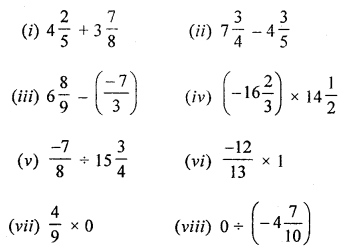Solution: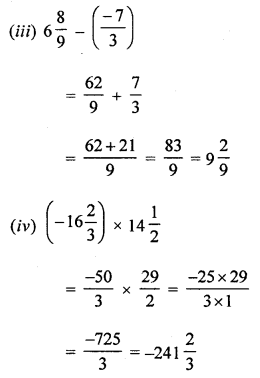Question 2.
What number should be added to $$\frac { -4 }{ 11 }$$ to get $$\frac { -3 }{ 8 }$$?
Solution:
Required number = $$\frac { -3 }{ 8 }$$ – ($$\frac { -4 }{ 11 }$$)Question 3.
What rational number should be subtracted from the sum of $$\frac { 3 }{ 14 }$$ and $$\frac { -4 }{ 7 }$$ to get $$\frac { 13 }{ 21 }$$?
Solution:Question 4.
If the product of two rational numbers is $$\frac { 25 }{ 42 }$$ and one of them -2$$\frac { 6 }{ 7 }$$, find the other.
Solution: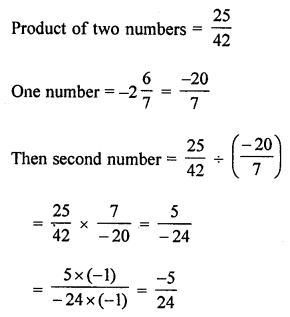Question 5.
Divide the sum of $$\frac { 4 }{ 13 }$$ and $$\frac { -3 }{ 2 }$$ by their product.
Solution:Question 6.
Using the appropriate properties of operations of rational numbers, evaluate the following:Solution: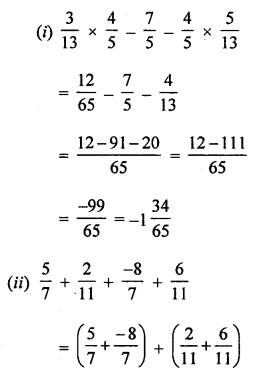Question 7.
Find the additive inverse of the following:
(i) -13$$\frac { 7 }{ 8 }$$
(ii) 4$$\frac { 3 }{ 6 }$$
Solution:
(i) Additive inverse of -13$$\frac { 7 }{ 8 }$$ is 13$$\frac { 7 }{ 8 }$$.
(ii) Additive inverse of 4$$\frac { 3 }{ 6 }$$ is -4$$\frac { 3 }{ 6 }$$.

Question 8.
Find the multiplicative inverse of the following:
(i) $$\frac { -23 }{ 46 }$$
(ii) 0
Solution:
(i) Multiplicative inverse of $$\frac { -23 }{ 46 }$$ is $$\frac { -46 }{ 23 }$$ = -2.
(ii) Multiplicative inverse of 0 is not defined.

Question 9.
Represent the following rational numbers on the number line:
(i) $$\frac { -3 }{ 11 }$$
(ii) $$\frac { 5 }{ 17 }$$
Solution:Question 10.
Insert five rational numbers between $$\frac { -3 }{ 7 }$$ and $$\frac { 2 }{ 5 }$$
Solution:
5 rational numbers between $$\frac { -3 }{ 7 }$$ and $$\frac { 2 }{ 5 }$$
LCM of 7, 5 = 35Question 11.
If p = $$\frac { -4 }{ 9 }$$ , q = $$\frac { 2 }{ 3 }$$ and r = $$\frac { -8 }{ 11 }$$, then verily the foflowing:
(i) p + (q + r) = (p + q) + r
(ii) p × q = q × p
(iii) p × (q + r) = p × q + p × r
(iv) (p + q) ÷ r = p ÷ r + q ÷ r.
Solution: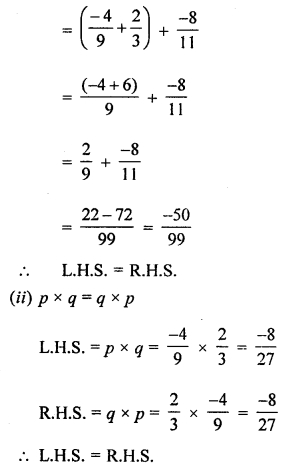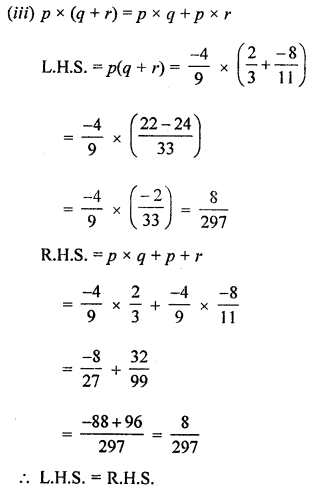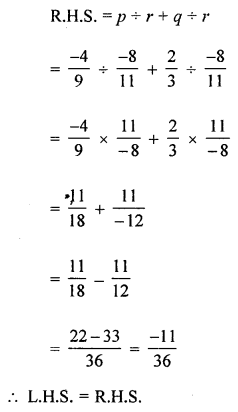Question 12.
A wedding cake weighed 8 kg. If $$\frac { 2 }{ 5 }$$ th of its weight was flour, $$\frac { 5 }{ 16 }$$ th was sugar, $$\frac { 1 }{ 4 }$$ thwas cream and the rest were nuts, find the weight of nuts.
Solution:
Weight of a wedding cake = 8 kg
Weight of Hour in it = $$\frac { 2 }{ 5 }$$ th of 8 kg = $$\frac { 16 }{ 5 }$$ kg